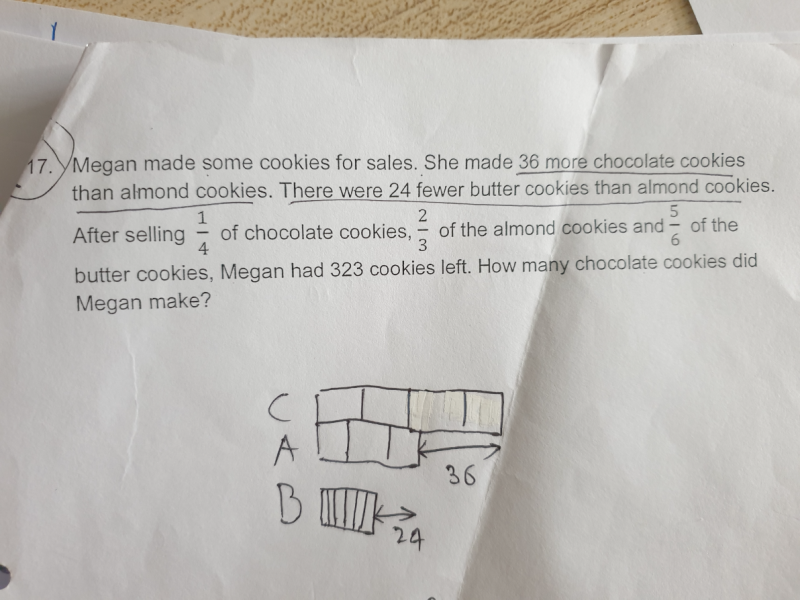# QuestionNeed help for this qns..

Thank you…

Alternative Solution:

Chocolate     [u]

Almond        [u]

Butter           [u]

(3/4) (u+60) + (1/3)(u+24) + (1/6)(u) = 323        <—— x 12 on both sides

9u + 540 + 4u + 96 + 2u = 3876

15u = 3240

u = 216

Hence number of chocolate cookies made by Megan = u + 60 = 216 + 60 = 276

C – A = 36 … (1)

A – B = 24 … (2)

(1/3)A + (1/6)B + (3/4)C = 323
A + (1/2)B + (9/4)C = 969 … (3)

(1) + (2):
C – B = 36 + 24 = 60 … (4)

(3) – (2):
(3/2)B + (9/4)C = 969 – 24 = 945
B + (2/3)(9/4)C = (2/3)945
B + (3/2)C = 630 … (5)

(4) + (5):
(5/2)C = 630 + 60 = 690
C = (2/5)690 = 276 #

PS. This is a crazy sum that requires 5 simultaneous equations!